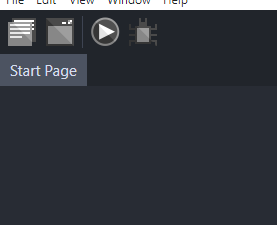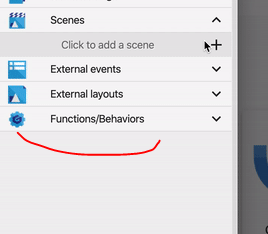# Hours and minutes in the same text using time string

my plan was to make a clock that had both hour and minutes on my game using the time string, but, it wont let me put them both on the same text, the method i used will result on a zero → /Time(“hour” + “min”)/ will result on a 0 in the text, and /Time(“hour”) + Time(“min”)/ results on a addition of those. is there a way i can achieve having both hours and minutes somewhat like this 00:00

update, it was just a question of rearanging the /modify the text/ event and using some other variables with the time string, and connect them to the text

Do you already know how to get hour and second as two serparate variable?
I don’t know how to get hour and second as variable, but if you can get that, then it should be just about displaying the two variables together.

Let’s say the variables are called Hour and Minute
Modify the text event would look like this: `(VariableString(Hour) + ":" + VariableString(Minute))`

But, we also want to add zero when either Hour or Minute is less than 10.
I think you can just make Hour or Minute variable a string by adding 0 in front of it.
Maybe: `"0" + VariableString(Hour) ` .

I have only done this in JavaScript, though. Here is a short example of the code for that:

```````  const currentTime = new Date();
hour = currentTime.getHours();
minute = currentTime.getMinutes();
second = currentTime.getSeconds();

if (hour < 10){hour = "0" + hour;}
if (minute < 10){minute = "0" + minute;}
if (second < 10){second = "0" + second;}
// If hours|minutes|seconds is less than 10 (less than 2 digits), put a 0 next to it

document.getElementById("time").innerHTML = String(hour)+serparator+String(minute);
// Here we actually change the span elements in the HTML using the variables to display the current time. `
``````

Hope it helps!

Yes! i figured it out today! using events it would go as the print right here, i will also implement those on the month segment

1 Like

Nice!
I suggest you put the first 4 events as sub-event that happens one time every second, using a Timer.
It should make your game a little faster when you add many things later.
For example, this is the event for score in my game:

If you just track seconds in a variable, there’s a pretty good extension that will display a variable as Hours/minutes/seconds in the main extension list.

could you please show how to do this

In the first button.Now I think it’s here